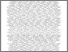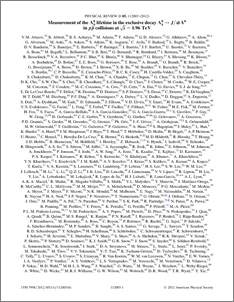# Measurement of the Lambda_b^0 lifetime in the exclusive decay Lambda_b^0 -> J/psi Lambda^0 in ppbar collisions at sqrt(s)=1.96 TeV

Collaboration, D0 and Bertram, Iain and Borissov, Guennadi and Fox, Harald and Ross, Anthony and Williams, Mark and Ratoff, Peter (2012) Measurement of the Lambda_b^0 lifetime in the exclusive decay Lambda_b^0 -> J/psi Lambda^0 in ppbar collisions at sqrt(s)=1.96 TeV. Physical Review D, 85 (11). ISSN 1550-7998Preview
PDF
PhysRevD.85.112003.pdf - Published Version

## Abstract

We measure the Lambda_b^0 lifetime in the fully reconstructed decay Lambda_b^0 -> J/psi Lambda^0 using 10.4 fb^-1 of ppbar collisions collected with the D0 detector at sqrt(s)=1.96 TeV. The lifetime of the topologically similar decay channel B^0 -> J/psi K^0_S is also measured. We obtain tau(Lambda_b^0) = 1.303 +- 0.075 (stat.) +- 0.035 (syst.) ps and tau(B^0) = 1.508 +- 0.025 (stat.) +- 0.043 (syst.) ps. Using these measurements, we determine the lifetime ratio of tau(Lambda_b^0)/tau(B^0) = 0.864 +- 0.052 (stat.) +- 0.033 (syst.).

Item Type:
Journal Article
Journal or Publication Title:
Physical Review D